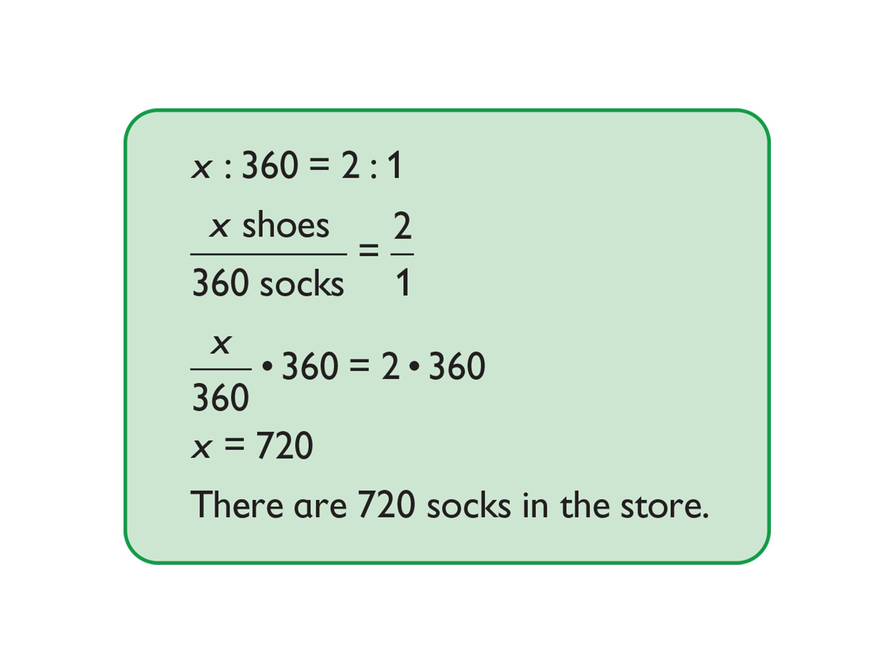# Multiplication Properties of Equality# Multiplication Property of Equality

The definition of the multiplication property of equality states:

If a = b, then ac = bc.

Discuss the following with your classmates.

• Where is the multiplication property of equality used in this example?
• How would you use a ratio table to solve this problem?1 of 8